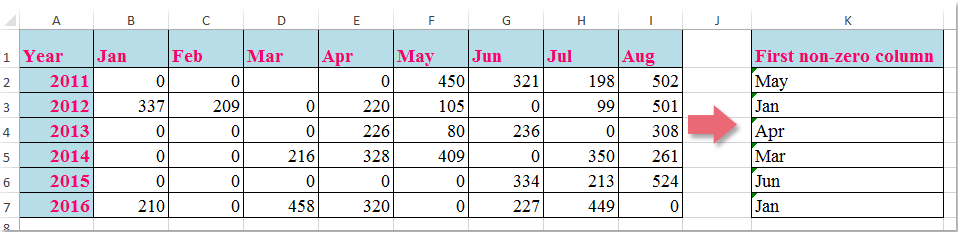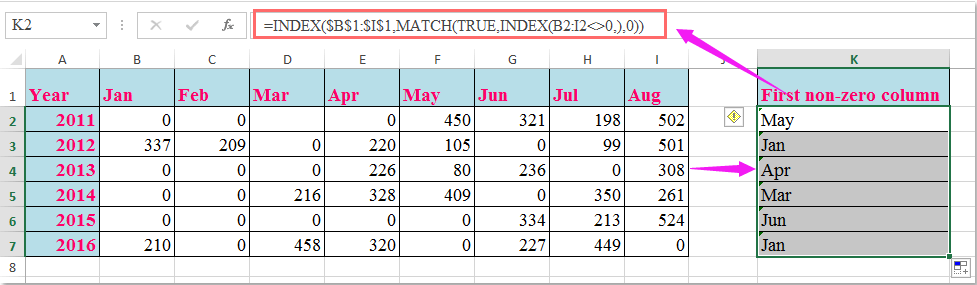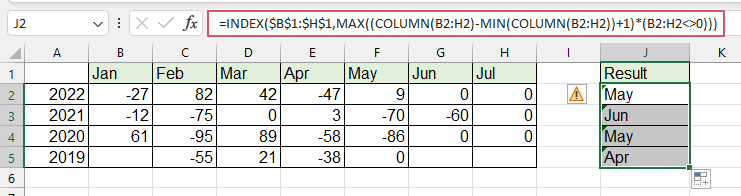## 如何在Excel中查找第一个非零值并返回相应的列标题？####查找第一个非零值，并使用公式返回相应的列标题### 最佳办公生产力工具

 热门特色: 查找、突出显示或识别重复项   |  删除空白行   |  合并列或单元格而不丢失数据   |   不使用公式进行四舍五入 ... 超级查询: 多条件VLookup  |   多值VLookup  |   跨多个工作表的 VLookup   |   模糊查询 .... 高级下拉列表: 快速创建下拉列表   |  依赖下拉列表   |  多选下拉列表 .... 列管理器: 添加特定数量的列  |  移动列  |  切换隐藏列的可见性状态  |  比较范围和列 ... 特色功能: 网格焦点   |  设计图   |   大方程式酒吧   |  工作簿和工作表管理器   |  资源库 （自动文本）   |  日期选择器   |  合并工作表   |  加密/解密单元格   |  按列表发送电子邮件   |  超级筛选   |   特殊过滤器 （过滤粗体/斜体/删除线...）... 前 15 个工具集:  12 文本 工具 (添加文本, 删除字符，...）   |   50+ 图表 类型 (甘特图，...）   |   40+ 实用 公式 (根据生日计算年龄，...）   |   19 插入 工具 (插入二维码, 从路径插入图片，...）   |   12 转化 工具 (小写金额转大写, 货币兑换，...）   |   7 合并与拆分 工具 (高级组合行, 分裂细胞，...）   |   ... 和更多#### Office Tab 为 Office 带来选项卡式界面，让您的工作更轻松

• 在Word，Excel，PowerPoint中启用选项卡式编辑和阅读，发布者，Access，Visio和Project。
• 在同一窗口的新选项卡中而不是在新窗口中打开并创建多个文档。
• 每天将您的工作效率提高50％，并减少数百次鼠标单击！No ratings yet. Be the first to rate!
This comment was minimized by the moderator on the site
Hello, my problem is: a have value 1,2,3,4,...50 that are the number of month. How to denormalize month into real datum. Thank you in advance a lot!!!
This comment was minimized by the moderator on the site
Does anyone know what you would do if your cell that contains a zero has a formula in it? When I use this formula, I end up with whatever the first column header is regardless of whether it is zero or not.
This comment was minimized by the moderator on the site
E como faço pra procurar o primeiro valor diferente de zero da esquerda pra direita?
This comment was minimized by the moderator on the site
Hello, Matheus
If you want to return the first non-zero value from a row, please apply the below formula:
=INDEX(B2:F2,MATCH(TRUE,INDEX(B2:F2<>0,),0))
This comment was minimized by the moderator on the site
What if you needed the last non-zero value in an array?

P.S. This was really helpful, thank you so much!
This comment was minimized by the moderator on the site
Hello, Ali,
To get the column header based on the last non-zero value, please apply the below formula, see screenshot:
=INDEX(\$B\$1:\$H\$1,MAX((COLUMN(B2:H2)-MIN(COLUMN(B2:H2))+1)*(B2:H2<>0)))
Please remember to press Ctrl+Shift+Enter key together.This comment was minimized by the moderator on the site
The formula is super helpful and helped me sorted out the situation! Thanks!!! Is there any chance you may please help to also explain how it works?
This comment was minimized by the moderator on the site
How do you look up for the second,third and fourth non-zero value?
This comment was minimized by the moderator on the site
Hello, Robert,
To lookup the second, third or fourth non-zero values and return their columns, please apply the below formula: (After pasting the formula, please press Ctr + Shift + Enter keys together.)
``=INDEX(\$B\$1:\$I\$1,SMALL(IF(B2:I2<>0, COLUMN(B2:I2)-COLUMN(A2)),2))``

Note: To extract the third or fourth result, you just need to change the number 2 to 3,4 as you need.

This comment was minimized by the moderator on the site
CAN U EXPLIAN HOW THIS FORMULA WORKS
This comment was minimized by the moderator on the site
Hello Priya,I am glad to help. I will separate the formula = INDEX(\$B\$1:\$I\$1,MATCH(TRUE,INDEX(B2:I2<>0,),0)) into 3 parts, and explain the formula to you step by step.
First, let us see the inner-most formula =INDEX(B2:I2<>0,). This formula returns an array of TRUE and FALSE whether the values of range B2:I2 are equal to 0. Please see the screenshot 1, formula =INDEX(B2:I2<>0,) returns {FALSE,FALSE,FALSE,FALSE,TRUE,TRUE,TRUE,TRUE}.
Second, =MATCH(TRUE,INDEX(B2:I2<>0,),0) =MATCH(TRUE, {FALSE,FALSE,FALSE,FALSE,TRUE,TRUE,TRUE,TRUE}, 0). It returns the position of the first value "TRUE", while 0 in the formula means an exact match. We can see that the first value "TRUE" occurs at the 5th position of the array. Then the second formula returns 5. See the screenshot 2.
Finally,  =INDEX(B1:I1,MATCH(TRUE,INDEX(B2:I2<>0,),0))=INDEX(B1:I1,5). It returns the 5th value of the array B1:I1. We can see in the screenshot that the value is "May". So the final formula returns "May".
PS: To drag the fill handle down the formula to get the rest of the results, we must make the range B1:I1 absolute. So we add \$ to range B1:I1 to keep it absolute.
Hope my explanation can solve your puzzle. Have a nice day.
Sincerely,Mandy
This comment was minimized by the moderator on the site
Thank you Mandy for illustrating it in detail.
Kindly guide how to get the last non zero value from same formula instead of first non zero value.
Will Match work for that or not?
This comment was minimized by the moderator on the site
Hi MandyZhou,

Thank you for the explanation!
I have a follow-up question.
How would the formula change if you had to look up the year too?
So in the above example, let's assume that column J is 'Year' and that in cell J2 is written '2015'.
And in cell K2 I would need the column header for the first non-zero value of the year in L2 (year 2015, so I would need to find 'Jun' in cell K2).